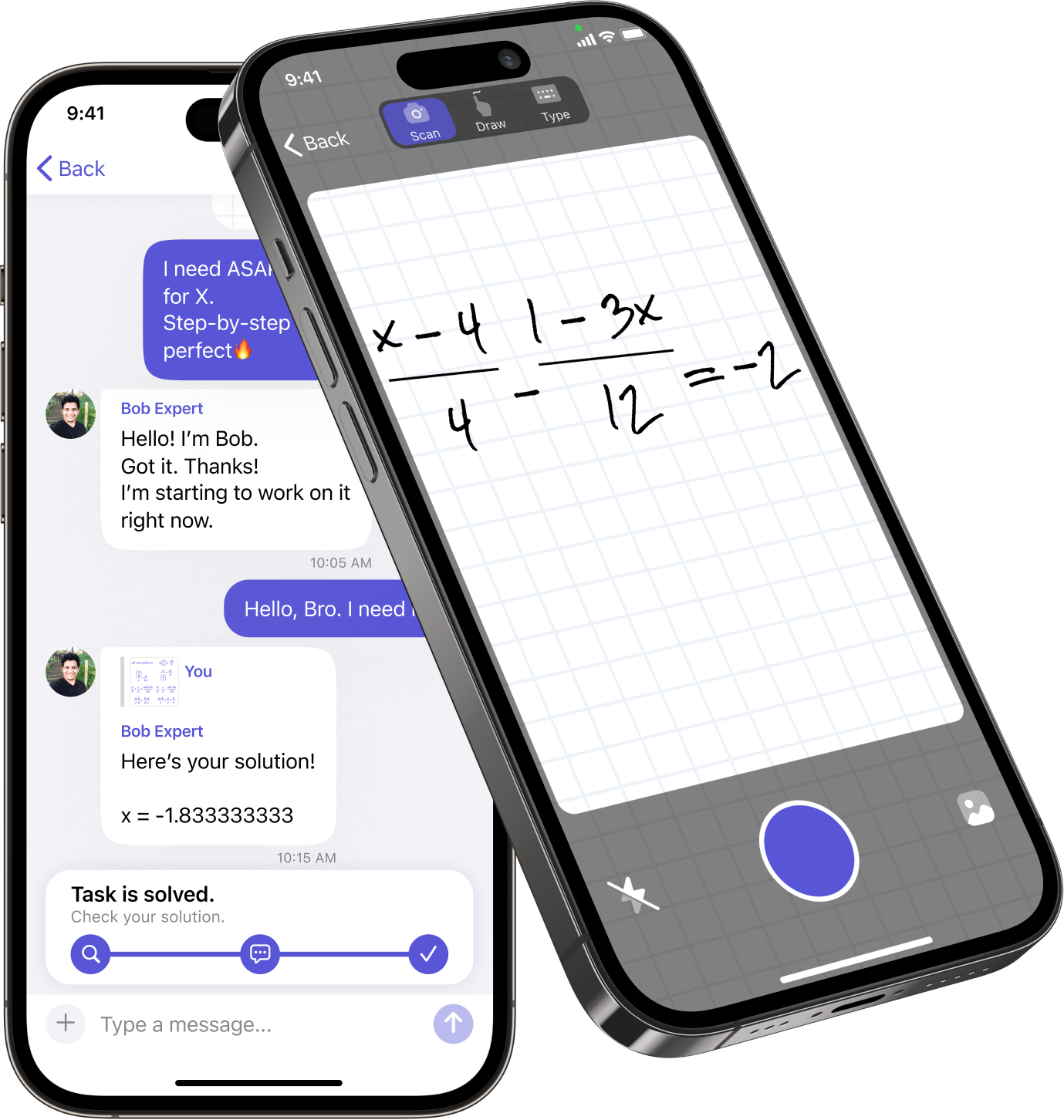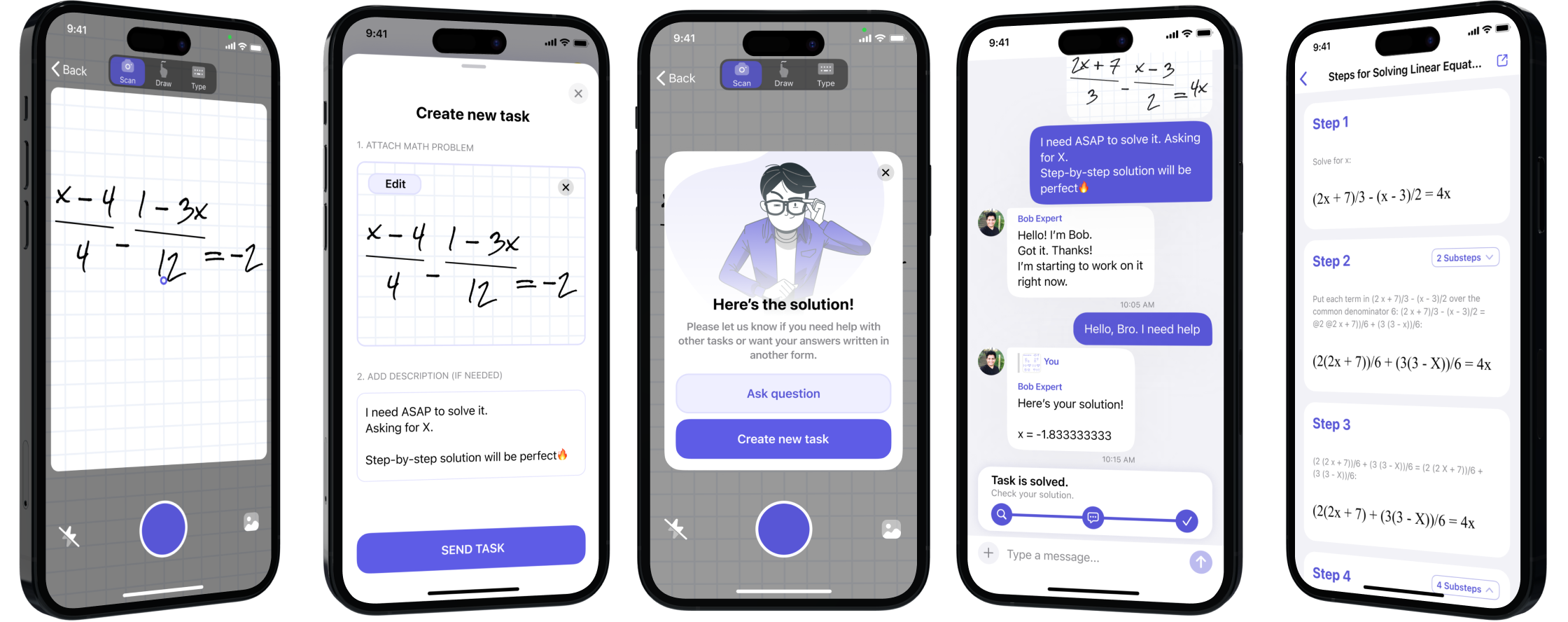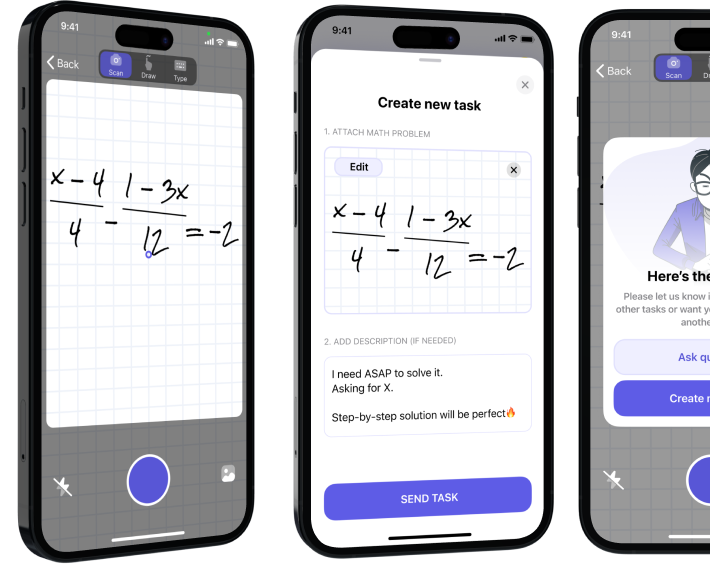Try supreme math solver enhanced by expert help from best math tutors online## MathMaster

is a math-solving and learning platform. It provides you with:

• step-by-step solution to any math task in a flash
• comprehensive help from real-person math experts in online chat 24/7

## Unique approach to math solving

01
Take a picture.
Snap your math task or input it manually with our smart calculator.
02
Solve.
03
Learn.
See solving steps and get detailed explanations from experts.## Instant help with all things mathExpert help in real-time .
Best math tutor assigned to you in online chat whenever you need.Knowledge base .
Learning materials, formula sheets, and quizzes to help you learn math easier.

Basic math
Arithmetic
High school math
Pre-algebra
Algebra
Geometry
Trigonometry
Statistics
Economics
Plane Geometry
Solid Geometry
Precalculus
Calculus
Functions
Trig functions

## Chat with experts, solve with confidenceDavid T.
Algebra • Trigonometry • Statistics • Solid Geometry • Calculus
Stanford UniversityMichael P.
Algebra • Trigonometry • Statistics
Massachusetts Institute of TechnologyEmily N.
Arithmetic • Calculus • Trigonometry
University of California, Los AngelesLily P.
Algebra • Trigonometry • Statistics • Solid Geometry • Calculus
University of Texas at AustinBenjamin K.
Algebra • Trigonometry • Statistics • Solid Geometry • Calculus
University of ChicagoMichael P.
Algebra • Trigonometry • Statistics
Massachusetts Institute of TechnologyEmily N.
Arithmetic • Calculus • Trigonometry
University of California, Los AngelesAndrew W.
Algebra • Trigonometry • Statistics • Solid Geometry • Calculus
University of California, BerkeleyBenjamin K.
Algebra • Trigonometry • Statistics • Solid Geometry • Calculus
Harvard UniversityMichael P.
Algebra • Trigonometry • Statistics
Massachusetts Institute of TechnologyEmily N.
Arithmetic • Calculus • Trigonometry
University of California, Los AngelesDaniel T.
Algebra • Trigonometry • Statistics • Solid Geometry • Calculus
University of Texas at Austin## Math solver trusted by MILLIONS of students worldwide

Leave your math tasks to MathMaster experts - we know the drill!10M +
users10k +1k +
math experts## Students love MathMaster

The easy-to-use interface, quick solutions, and expert chat have boosted my confidence in tackling math problems. Two thumbs up!
Mary
Student
The best math calculator ever, that's for sure! There's a scanner that identifies any task and solves it superfast.
Ann
Student
MathMaster is a game-changer! It's like having a personal math tutor in my pocket. The step-by-step explanations help me understand the concepts better. Highly recommended!
Albert
Student
The step-by-step explanations help me understand the concepts better. Highly recommended!
John
Student
The scanner recognizes problems instantly, and the step-by-step solutions make learning a breeze. I wish I had this app earlier!
Peter
Student
MathMaster is my secret weapon for acing math tests.
Marta
Student